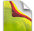### Feel The Power# Javascript Functions

Functions are the central working units of JavaScript. Nearly every script you'll write uses one or more functions. Therefore it is important that you understand what a function is and how it works.

First I explain the basic syntax, of a function, then I explain how to call it. After that you learn how to pass arguments and why you should do this. Finally, we make the function return something.

### The function

This is a function:

``````function example(a,b) {
number += a;
alert('You have chosen: ' + b);
}``````

It is called like this:

``example(1,'house')``

In fact, when you define the function `example` you create a new JavaScript command that you can call from anywhere on the page. Whenever you call it, the JavaScript inside the curly brackets `{}` is executed.

### Calling the function

You can call the function from any other JavaScript. After the function is executed, the other script that called it goes on from the call.

``````alert('Example 1: the House');
example(1,'house');
(do more stuff)``````

So this script first generates an alert box, then calls the function and after the function is finished it continues to do the rest of the instructions in the calling code.

### Arguments

You can pass arguments to a function. These are variables, either numbers or strings, with which the function is supposed to do something. Of course the output of the function depends on the arguments you give it.

In the example we pass two arguments, the number `1` and the string '`house`':

``example(1,'house');``

When these arguments arrive at the function, they are stored in two variables, `a` and `b`. You have to declare this in the function header, as you can see below.

``function example(a,b)``

Now the function starts working and does something with a and b:

``````function example(a,b) {
number += a;
alert('You have chosen: ' + b);
}``````

It adds 1 to `number` and alerts: You have chosen: house. Of course, if you call the function like

``example(5,'palace');``

it adds 5 to `number` and alerts You have chosen: palace. The output of the function depends on the arguments you give it.

#### Using more arguments

You can use as many arguments as you like:

``````function example(a,b,c,data,data2) {
number += a;
alert('You have chosen: ' + b);
(do lots more with c, data and data2)
}``````

If you forget to pass one argument the function complains. Suppose you do

``example(1,'house',16,'more stuff')``

then 1 is passed to `a`, 'house' is passed to `b`, 16 is passed to `c` and 'more stuff' is passed to `data`. But now there is nothing for `data2` since you did not pass anything to it in the function call. When the function arrives at the part it is supposed to do something with `data2`, it finds that `data2` does not exist and gives error messages.

So always be careful to pass enough arguments. Personally I always try to write functions that need as little arguments as possible. This keeps my code clean and makes updating easy.

#### Using no arguments

You can also write a function without any arguments:

``````function example() {
number += 1;
}``````

Of course the function does exactly the same whenever you call it. If you try to give it arguments, it ignores them since they are not defined in the function header.

### Returning a value

One more thing a function can do is return a value. Suppose we have the following function:

``````function calculate(a,b,c) {
d = (a+b) * c;
return d;
}``````

This function calculates a number from the numbers you pass to it. When it's done it returns the result of the calculation. This is in fact the opposite of passing arguments: the function now passes the result back to its calling handle. After any `return` the function stops working: it doesn't execute any more lines, if there are any.

In practice this works as follows:

``````var x = calculate(4,5,9);
var y = calculate((x/3),3,5);``````

Now you declare a variable `x` and tell JavaScript to execute `calculate()` with the arguments 4, 5 and 9 and to put the returned value (81) in `x`. Then you declare a variable `y` and execute `calculate()` again. The first argument is `x/3`, which means 81/3 = 27, so y becomes 150.

Of course you can also return strings or even Boolean values (`true` or `false`). When using JavaScript in forms, you write a function that returns either `true` or `false` and thus tells the browser whether to submit a form or not.

#### Returning to stop the function

Sometimes it's useful to add a `return` to your script to stop the script. If you use object detection and you find out the browser can't handle a certain script, use a `return` to end the function before the browser reaches the dangerous lines it can't handle.

Suppose you write a nice W3C DOM script. The first thing you'd have to do is seeing if the browser supports the W3C DOM:

``````function initPage() {
var supported = document.createElement && document.getElementsByTagName;
if (!supported) return;
// start of DOM script
var x = document.getElementById('writeroot');
// etc.
}``````

If `supported` is false (browser doesn't support the W3C DOM), the function stops (it would only lead to error messages in this browser). You do this by using `return`. Only if the browser supports the W3C DOM it makes it to the next line where the actual DOM script starts.

#

#

#

### Javascript Functions Tutorial

TOP TUTORIALS
WHAT IS NEW IN KERIXA?
RECENT TUTORIALS
CATEGORIES